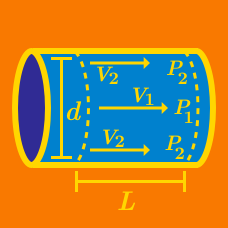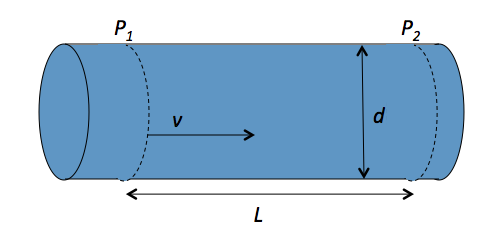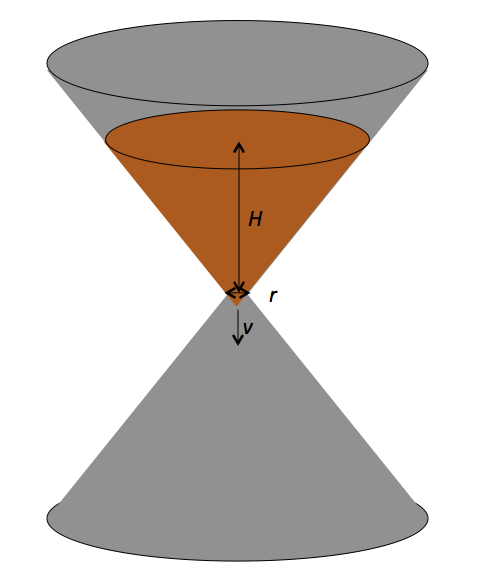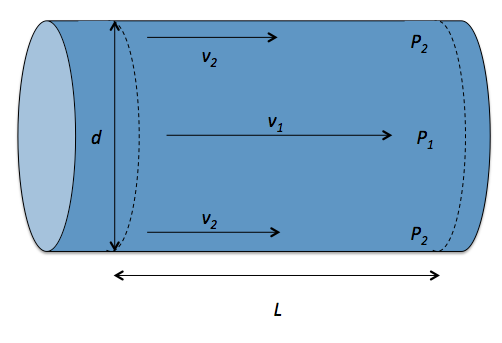Classical Mechanics

# Buckingham Pi Theorem (Dimensional Analysis)

Using the Buckingham $$\pi$$ theorem, find the formula for the radius of a black hole in term of the black hole’s mass $$m,$$ the gravitational constant $$G,$$ and the speed of light $$c$$As shown in the above figure, a liquid with density $$\rho$$ and viscosity $$\mu$$ flows through a pipe with diameter $$d.$$ In a section of the pipe with length $$L,$$ the liquid flows with a speed of $$v.$$ Using the Buckingham $$\pi$$ theorem, determine the pressure difference $$\Delta P = P_1 - P_2$$ in terms of the fluid properties $$d, L, \rho, \mu$$ and $$v .$$

Details

• the function $$f$$ is unitless, so that it outputs a pure number.The sand timer in the above figure has a due time of $$T.$$ The radius of the hole is $$r,$$ the initial height of the sand is $$H,$$ and the density of the sand is $$\rho.$$ Using the Buckingham $$\pi$$ theorem, determine $$T$$ in terms of the properties $$r, H, \rho,$$ and the gravitational constant $$G .$$As shown in the above figure, a liquid with density $$\rho$$ flows through a pipe with diameter $$d.$$ In a section of the pipe with length $$L,$$ the liquid flows with a speed of $$v_1$$ at the center and $$v_2$$ at the edge of the flow. The pressures are $$P_1$$ at the center and $$P_2$$ at the edge. Using the Buckingham $$\pi$$ theorem, express the viscosity $$\mu$$ of the fluid in terms of the liquid properties $$d, L, \rho, \Delta v = v_1 - v_2$$ and $$\Delta P = P_1- P_2 .$$

The drag force $$F$$ depends on four quantities: two parameters of the cone which are the speed of the cone $$v$$ and the size of cone $$r,$$ and two parameters of the air which are the density of the air $$\rho$$ and the viscosity of the air $$\mu.$$ Find the independent dimensionless groups that can be produced with $$F, v, r, \rho$$ and $$\mu .$$

×

Problem Loading...

Note Loading...

Set Loading...